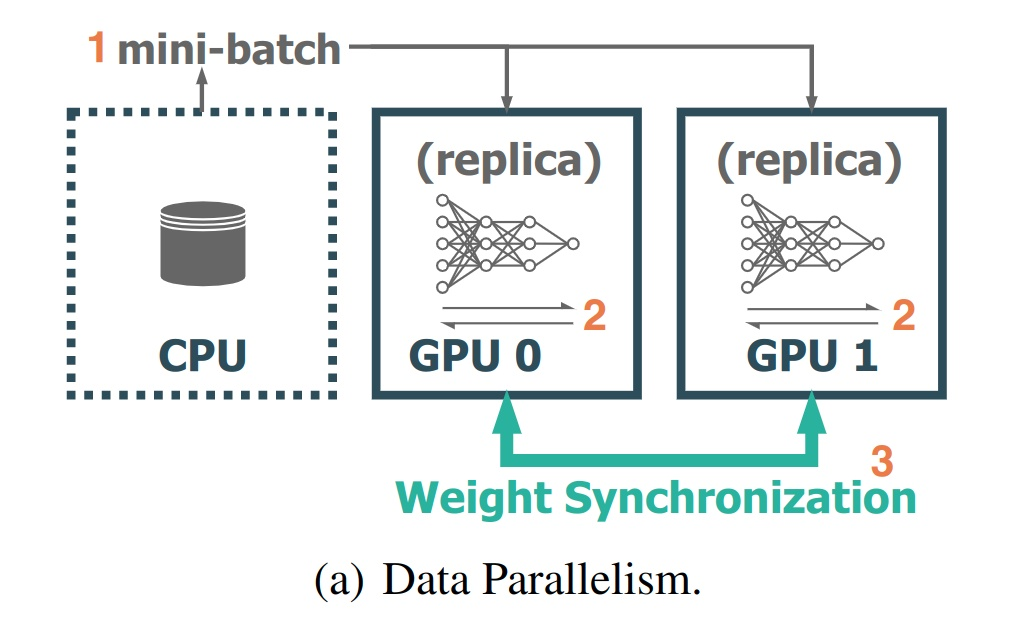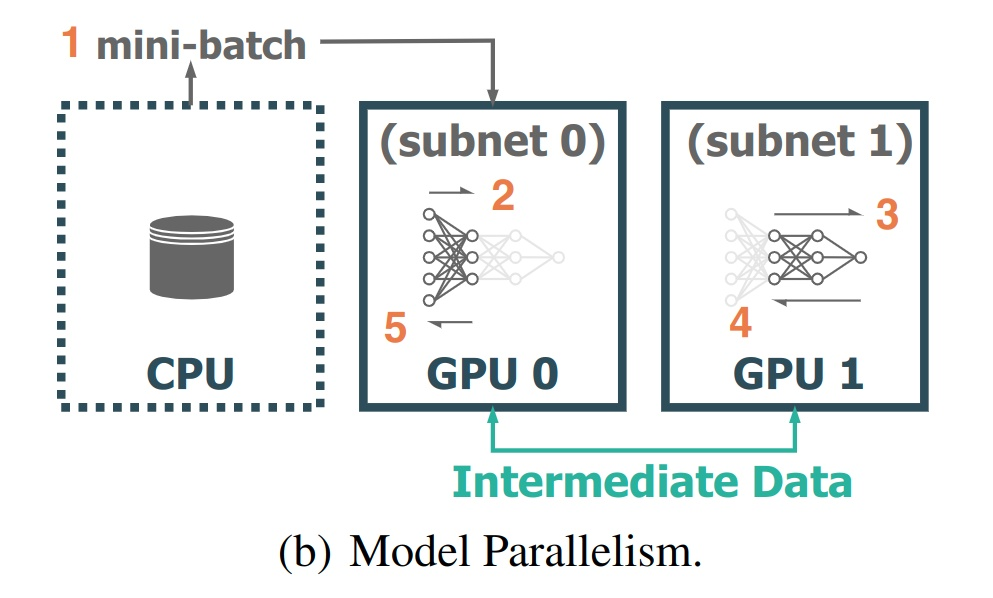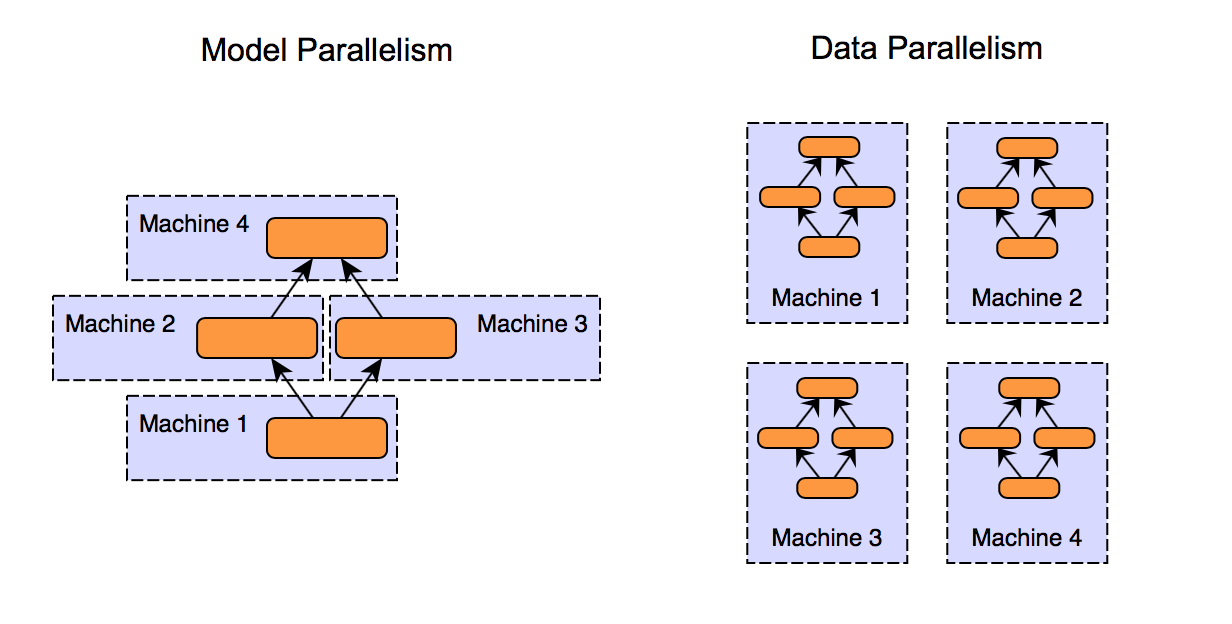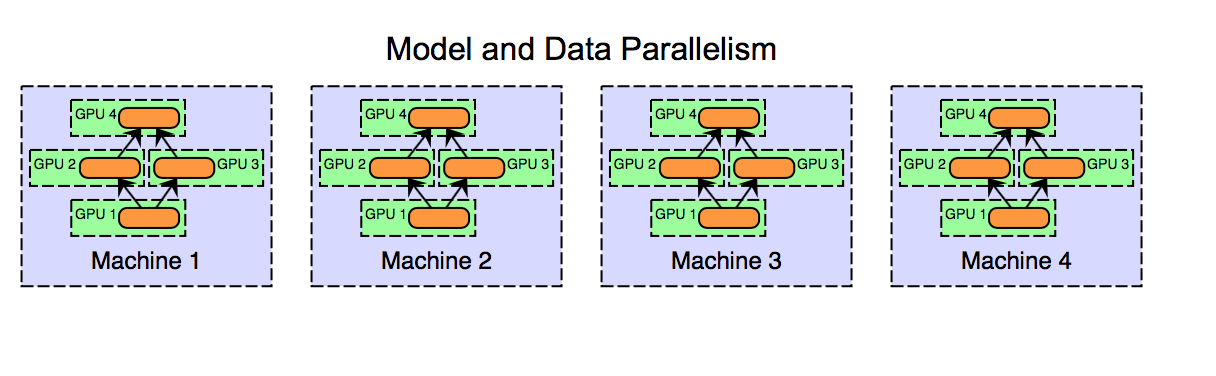# 分布式深度学习简介：数据并行和模型并行

## 前言

1. 数据并行 (Data Parallel)
2. 模型并行 (Model Parallel)
3. 数据模型并行（Data-Model Parallel）

## 数据并行数据并行

\begin{aligned} \frac{\partial L}{\partial w} &= \frac{\partial \Big[ \frac{1}{n} \sum_{i=1}^{n} f(x^{(i)}, y^{(i)}) \Big] }{\partial w} \\ &= \frac{1}{n} \sum_{i=1}^{n} \frac{\partial f(x^{(i)}, y^{(i)}) }{\partial w} \\ &= \frac{m_1}{n} \frac{\partial \Big[ \frac{1}{m_1} \sum_{i=1}^{m_1} f(x^{(i)}, y^{(i)}) \Big] }{\partial w} + \cdots + \frac{m_k}{n} \frac{\partial \Big[ \frac{1}{m_k} \sum_{i=m_{k-1} + 1}^{m_{k-1} + m_k} f(x^{(i)}, y^{(i)}) \Big] }{\partial w} \\ &= \frac{m_1}{n} \frac{\partial l_1}{\partial w} + \frac{m_2}{n} \frac{\partial l_2}{\partial w} + \cdots + \frac{m_k}{n} \frac{\partial l_k}{\partial w} \end{aligned}

\begin{aligned} \frac{\partial L}{\partial w} &= \frac{1}{k} \big[ \frac{\partial l_1}{\partial w} + \frac{\partial l_2}{\partial w} + \cdots + \frac{\partial l_k}{\partial w} \big] \end{aligned}

## 模型并行模型并行

## 一些思考模型并行和数据并行数据模型并行